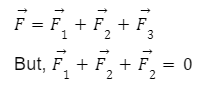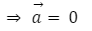• Call Now

1800-102-2727•

## Newton’s First Law Of Motion: Definition, Inertia And Mass, Types Of Inertia

Let’s say that you want to roll a ball on a very long horizontal surface with constant velocity. Is force required to apply on the ball throughout the motion? You might say yes, as because of external opposing forces such as friction or viscous force the velocity of the ball will keep decreasing and after some time the ball will come to rest.  Now let’s consider an ideal situation such that the surface is frictionless and there are no opposing forces on the ball. In that case the ball will continue to move with its constant velocity for infinite time. This situation is explained by Newton’s first law of motion. In this article let’s understand this law in detail.

## Newton’s First Law of Motion

In the movies, you must have observed that if a person jumps from a moving car he/she will always fall in the direction of the car. This situation is explained by Newton's first law of motion.

It states that in an inertial frame of reference a body remains in the state of rest or uniform motion in a straight line unless and until an external force acts on it.

In the figure below, the resultant force is zero, hence the object remains unaccelerated.
We can write net force on an object as,In simple words Newton’s 1st law of motion says that a body will not start moving until and unless we apply external force on it. If the body is in motion, it will not stop or change its velocity until and unless some external force is applied.

## Inertia and Mass

The resistance of a particle to change its state of rest or of uniform motion along a straight line is called inertia.

Note: First law is also known as the law of inertia.

Physical Quantification of Inertia

For translational motion, the body having more mass will have more inertia. Hence, mass is said to be a measure of inertia.

## Types of Inertia

There are three types of inertia:### Inertia of Rest

It is the inability of a body by virtue of which it can not move by itself. A body at rest remains at rest and can not start moving on its own due to inertia.

Example:

• If you are sitting in a bus, you fall backward when the bus starts suddenly. This is because the sudden start of the bus brings motion to the bus and to our feet in contact with the bus. But the rest of our body opposes this motion because of inertia so they fall backward.

### Inertia of Motion

It is the inability of a body that is in motion to stop by itself. A body in uniform motion does not get accelerated or retarded on its own. It also can not come to rest on its own.

Example:

• A person sitting in a bus falls forward when the bus stops suddenly. This is because the sudden stopping of the bus brings motion of the bus as well as our feet in contact with the floor of the bus to rest. But the rest of our body is in motion because of inertia so they fall forward.

### Inertia of Direction

It is the inability of the body by virtue of which it can not change its direction of motion by itself.

Example:

• When the bus makes a sharp turn at high speed, the driver and passengers tend to get thrown to the other side due to directional inertia.## Practice Problems of Newton's First Law of Motion

Question 1. Which of the following has higher inertia: Eraser or Notebook?

Answer: Notebook will have higher inertia than eraser because notebook has more mass than eraser.

Question 2. Is an external force necessary to keep a body in uniform motion? Justify your answer.

Answer: External force is not at all necessary to keep the body in uniform motion. According to Newton’s second law a body which is in uniform motion will continue to move in uniform motion unless unbalanced force acts on it.

Question 3. A notebook lying on a desk doesn't start moving without an external force, why?

Answer: Because of the inertia of the rest of a notebook lying on a desk it remains at rest.

Question 4.What is physical quantification of inertia?

Answer: For translational motion, the body having more mass will have more inertia. Therefore, mass is a measure of inertia.

## FAQs of Newton's First Law of Motion

Question 1. What is another name for Newton's first law of motion ?

Answer: Newton's first law of motion is also called the law of inertia.

Question 2. The only way to speed up a moving object is to apply a net force to it. [True/False]

Answer: True. According to Newton's first law of motion we can slow down a moving object only by applying force in the opposite direction.

Question 3. What is Inertia?

Answer: The resistance of a particle to change its state of rest or of uniform motion along a straight line is called inertia.

Question 4. What are the types of inertia?

Answer: There are three types of inertia : inertia of rest, inertia of motion, inertia of direction.

## NCERT Class 11 Physics Chapters

 Physical World Units and Measurements Motion in a Straight Line Motion in a Plane Laws of Motion Work Energy and Power Particles and Rotational Motion Gravitation Mechanical Properties of Solids Mechanical Properties in Liquids Thermal Properties of Matter Thermodynamics Kinetic Theory Oscillations WavesTalk to our expert
Resend OTP Timer =
By submitting up, I agree to receive all the Whatsapp communication on my registered number and Aakash terms and conditions and privacy policy